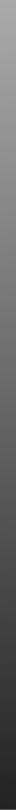Overview
Forum
Mango
iMango
Papaya
Multi-image Analysis GUI

## Color Table Specification

The Add/Edit Color Table feature of Mango can be used to import/export color tables in the below format. Files are saved with an extension of .lut, but this is not necessary for the format.

The following is a basic example and describes a spectrum with black on the low end and white on the high end.

# The name of the color table
Name=MyColorTable

# The number of knots used in this color table
NumberOfKnots=7

# List of knots, starting with zero.
# Each knot consists of five space-delimited values:
# Location - a decimal value from 0 to 1 - the placement of the knot along the color table continuum
# Red - an integer value from 0 to 255 - the degree of red component
# Green - an integer value from 0 to 255 - the degree of green component
# Blue - an integer value from 0 to 255 - the degree of blue component
# Gradient - a boolean value of 0 or 1 - if 1, then colors will change continuously between this knot and the next
Knot0=0.0 0 0 0 1
Knot1=0.1 0 0 255 1
Knot2=0.33 0 255 255 1
Knot3=0.5 0 255 0 1
Knot4=0.66 255 255 0 1
Knot5=0.9 255 0 0 1
Knot6=1.0 255 255 255 0

Another type of color table specifies an expression. This expression describes the change in color values from the lower knot to the higher knot (only two knots can be used in this case). The keyword value is used to indicate the location along the continuum from 0 to 255. The expression should return a decimal value from 0 to 1, indicating the strengths of the lower and higher knots at each location along the continuum. A value of 0 indicates the lower knot is used 100%. A value of 1 indicates the higher knot is used 100%. The following is an example of this type of color table.

# The name of the color table
Name=MyExpressionColorTable

# The number of knots used in this color table
NumberOfKnots=2

# List of two knots
Knot0=0.0 0 0 0 1
Knot1=1.0 255 255 255 0

# Expression
Expression=(1 - pow(2.71828, -5 * value)) / (1 - pow(2.71828, -5))